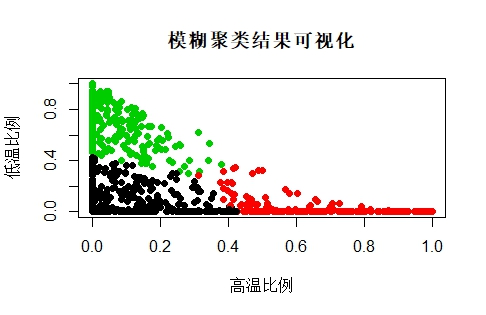# r语言模糊聚类（真实案例完整流程）机器学习 同时被 2 个专栏收录22 篇文章 1 订阅

## 算法介绍：

**概念：**模糊聚类分析是根据客观事物间的特征、亲疏程度、相似性，通过建立模糊相似关系对客观事物进行聚类的分析方法【搜狗百科】

**模糊聚类分析：**模糊识别又称为模糊分类。从处理问题的角度来看，模糊识别可以分为有监督的分类和无监督的分类两种类型。在模糊理论中，一个元素与一个集合的关系不再是简单的属于或者不属于关系。而是属于，不属于，部分属于。

FCM算法简介： FCM算法是基于对目标函数的优化基础上的一种数据聚类方法。聚类结果是每一个数据点对聚类中心的隶属程度，该隶属程度用一个数值来表示。FCM算法是一种无监督的模糊聚类方法，在算法实现过程中不需要人为的干预。FCM的C跟K-Means的K是一样的，指的是聚类的数目。F(Fuzzy)是模糊的意思，指的是”一个事件发生的程度“。

• 需要设定一些参数，若参数的初始化选取的不合适，可能影响聚类结果的正确性；
• 当数据样本集合较大并且特征数目较多时，算法的实时性不太好

1. 建立数据矩阵；
2. 数据标准化：因为在实际的问题中，不同的数据可能有不同的量纲，为了能够比较，通常进行数据规格化。常用的方法有标准差标准法，极差正规化，最大值规格化；
3. 建立模糊相似矩阵，初始化隶属矩阵：求出x(i)与x(j)的相似关系矩阵，常用的方法有相似系数法，距离法，贴近度法，余弦法；
4. 算法开始迭代，直到目标函数收敛到极小值；
5. 根据迭代结果，由最后的隶属矩阵确定数据所属的类，显示最后的聚类结果。

## R语言实现

https://blog.csdn.net/yawei_liu1688/article/details/79644364

setwd("E:/data")
data <- data_model[,2:4]


min.max.norm <- function(x){
((x-min(x))/(max(x)-min(x)))
}

data <- apply(data,2,min.max.norm)


FCM <- function(x, K, mybeta = 2, nstart = 1, iter_max = 100, eps = 1e-06) {

## modified time: 2018-03-23

FCM_onetime <- function(x, init_centers, mybeta = 2, iter_max = 100, eps = 1e-06) {
n = dim(x)
d = dim(x)
g = init_centers
K = dim(g)
histJ = c()
pasfini = 1
Jold = Inf
D = matrix(0, n, K)
for (j in 1:K) {
D[, j] = rowSums(sweep(x, 2, g[j, ], "-")^2)
}
iter = 1
J_old = Inf
while (pasfini) {
s = (1/(D + eps))^(1/(mybeta - 1))
u = s/(s %*% matrix(1, K, K))
t1 = t(u^mybeta) %*% x
t2 = t(u^mybeta) %*% matrix(1, n, d)
V = t1/t2
g = V
D = matrix(0, n, K)
for (j in 1:K) {
D[, j] = rowSums(sweep(x, 2, g[j, ], "-")^2)
}
J = sum(u^mybeta * D)
pasfini = abs(J - Jold) > 0.001 && (iter < iter_max)
Jold = J
histJ = c(histJ, J)
iter = iter + 1
}
cluster_id = apply(u, 1, which.max)
re = list(u, J, histJ, g, cluster_id)
names(re) = c("u", "J", "histJ", "g", "cluster_id")
return(re)
}
x = as.matrix(x)
seeds = 1:nrow(x)
id = sample(seeds, K)
g = as.matrix(x[id, ])
re_best = FCM_onetime(x = x, init_centers = g, mybeta = mybeta, iter_max = iter_max, eps = eps)
if (nstart > 1) {
minJ = 0
i = 2
while (i <= nstart) {
init_centers_id = sample(seeds, K)
init_centers = as.matrix(x[init_centers_id, ])
run = FCM_onetime(x, init_centers = init_centers, mybeta = mybeta, iter_max = iter_max)
if (run$J <= re_best$J) {
re_best = run
}
i = i + 1
}
}
return(re_best)
}


• x: 输入矩阵 n*d, n d-dim 样本 ；
• K: 簇的数量
可选 :
• mybeta : FCM目标函数中控制隶(u)属度平滑程度的变量。默认值2；
• nstart: 随机组数量（默认1） ；
• iter_max : 允许的最大迭代次数（默认100）

• u: 模糊隶属度矩阵 = n * K的矩阵；
• g: K * d矩阵的簇中心；
• J: 目标函数；
• histJ: iter进程中的所有目标函数值

result <- FCM(data,K=3) #进行聚类
result

#聚类可视化
plot(data_model$V1,data_model$V3,col=result$cluster_id,main="模糊聚类结果可视化",xlab='高温比例',ylab='低温比例',pch=19)聚类结果输出并保存： result_output_fcm <- data.frame(data_model[,1:4],result$cluster_id)
write.csv(result_output_fcm,file="result_output_fcm.csv",row.names=T,quote=F)

09-2501-27
12-093666
05-2391
08-053046
01-28
04-03
04-096万+
01-031119
04-13
03-01
05-071万+
12-08455
04-276161
05-29点击重新获取扫码支付余额充值